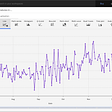# Solving Common Probability Problems with Python Pt.1 — Binomial

## Scenario One:

`from scipy import statsimport matplotlib.pyplot as pltimport pandas as pd# Parameterize the case. Variable names are self explained. total_trial = 5yes_odds = 1 / 6num_of_yes = 3culm_less_equal = num_of_yes - 1# Declare the statistics binom instancebinom = stats.binom(total_trial, yes_odds)# Compute the probability of cumulative density of less and equal to success number of ONE(s). (0, 1, 2 times)less_equal_cdf = binom.cdf(culm_less_equal)# Remaining is cumulative of greater and equal than 3 ONEs. (3, 4, 5 times)greater_cdf = 1 - less_equal_cdfprint(f"You have {round(greater_cdf * 100, 2)}% chance of having at least {num_of_yes} x ONEs. Good luck!")# (optional below) Graph it. # We want individual probability outcomes as list values. # pmf() to get individual data point.# Declare and initialize the empty data variable. pmf_dict = {    "xtimes": [],    "probability": []}# Compute exact probability of 0xONE, 2xONE, 3xONE, ... so forth to 5xONE.# add them to pmf dictionary. Using probability mass function (PMF)for i in range(6):    pmf = binom.pmf(i)    pmf_dict["xtimes"].append(i)    pmf_dict["probability"].append(pmf)# Plot. visualize the datadf = pd.DataFrame(pmf_dict)print(df)df.plot.bar(y="probability", x="xtimes")plt.show()# Simulation (optional) of counting test. # Test for ever 100 rounds: how many success events (more than 3 ONEs) we have.  do 10 x 100 rounds.print("\n --- Simulation Starts --- \n")for i in range(10):    event_count = 0    simulated = binom.rvs(100)    for j in range(100):        if simulated[j] >= 3:            event_count += 1    print(f"{event_count} TIMES of having at least three ONEs in 100 rounds.")    print("\n --- Simulation Ends --- \n")`

## Scenario Two:

`from scipy import statsimport matplotlib.pyplot as pltimport pandas as pd# Parameterize the case. total_trial = 5yes_odds = 1 / 6# Geometric Distribution instancegeom = stats.geom(yes_odds)# Declare the empty data variable.pmf_dict = {    "num_of_trial": [],    "probability": []}for i in range(total_trial):    pmf_dict["num_of_trial"].append(i + 1)    pmf_dict["probability"].append(geom.pmf(i + 1))    # Make the DataFrame instance.df = pd.DataFrame(pmf_dict)print(df)# Plotdf.plot.bar(x="num_of_trial")plt.show()# Get the cumulative probabilitycdf = geom.cdf(5)print(f"\nFor each round(5 rolling), we have {round(cdf * 100, 2)}% chance of having a ONE.")`
• to have a ONE showing in the very first rolling is 16.67% which is very intuitive 1/6.
• To have a ONE first time showing in the very 2nd rolling is 13.89%,
• in 3rd 11.56%, in 4th 9.65%, in 5th 8.04%.

--

--

--

## More from Weiming Chen

I occasionally write about software, web, blockchain, machine learning, random thoughts.

Love podcasts or audiobooks? Learn on the go with our new app.

## Learn computational science quickly## Top Python Libraries for Visualization: A Starting Guide## The Statistics Behind James Corden’s “Know For Your Row” Game Segment## Supercharging your Mobile Apps: GPU Accelerated Machine Learning using Android NDK & Vulkan Kompute## A Simple Multiprocessing Framework Within Python## Forecasting min temperatures with AutoAI missing imputation## Functional “Control Flow” — Writing Programs without Loops## What lies behind YouTube Search infinite results## Weiming Chen

I occasionally write about software, web, blockchain, machine learning, random thoughts.

## Turning Raw Poultry Farm Data into meaningful insights.## How to Set X and y in Pandas## Data Analytics — Week 1